• matlab基本命令 复数 a=real(z) %计算实部 b=imag(z) %计算虚部 r=abs(z) %计算幅值 theta=angle(z) %计算相角 angle(a)*180/pi %以角度为单位计算相角 数值矩阵 #生成 from:to %默认step=1 from:step:to linspace...
环境功能
M文件即脚本、多行命令 MAT文件即数据文件，用于储存变量 上下键于命令窗口为复现上下行
matlab基本命令
复数
a=real(z) %计算实部
b=imag(z) %计算虚部
r=abs(z) %计算幅值
theta=angle(z) %计算相角
angle(a)*180/pi %以角度为单位计算相角


数值矩阵
#生成
from:to %默认step=1
from:step:to
linspace(a,b,n) %a到b线性分布n个元素组成向量

#特殊
zeros(m,n)
ones(m,n)
rand(m,n)  %均匀分布随机
randn(m,n) %正态分布随机
eye(m,n)   %单位矩阵

#提取与赋值（超出则填0）
a(i,j) %全下标
a(i)   %长列单下标

# 子矩阵产生与赋值
a([1 3],[2 3])%取行数为 1、3，列数为 2、3 的元素构成子矩阵
a(1:3,2:3)    %1~3，2~3
a(:,3)        %取第三列
a(1:3,end)    %end表最大值
a([1 3;2 6])  %单下标生成
#逻辑矩阵提取例
b=a>1 %得出逻辑向量 b
b =
0 1 0
1 1 0
1 1 1
a(b) %按单下标顺序排成长列
ans =
3
5
2
4
6
9
#删除直接赋值[]
#可利用[]直接连接矩阵



字符串
str1='Hello'           %单引号表示
str4=[str1,'! ',str2]  %连接字符串，按照列连接必须大小相同
str8=strvcat(str1,str2)%以列相接
eval(str9)             %执行


矩阵运算
det(X)        %计算方阵行列式
rank(X)       %求矩阵的秩
inv(X)        %求矩阵的逆阵
[v,d]=eig(X)  %计算矩阵特征值和特征向量
diag(X)       %产生X矩阵的对角阵
[l,u]=lu(X)   %方阵分解为一个准下三角方阵和一个上三角方阵的乘积
[q,r]=qr(X)   %矩阵分解为一个正交方阵 q 和一个与X同阶的上三角矩阵 r 的乘积
[u,s,v]=svd(X)%分解为三个矩阵的乘积，其中 u,v 为正交方阵，s 为对角阵（奇异值）



矩阵数组算数运算
± 若为标量则表示分别 *、.*点乘为对应相乘/\，/.\同样 解线性方程显示特解或最小二乘解 矩阵乘方的运算表达式为“A^B”，其中 A 可以是矩阵或标量。搜索关键词看情况 A’表转置，负数矩阵为共轭转置，A.'表示数组 A 的转置，如果数组 A 为复数数组，则不是共轭转置ceil为+=
展开全文• 复数矩阵求逆运算C++代码，可以准确的求其结果！
• 对常用的矩阵运算，函数，作图等应用的总结！对拟合工具的使用作了详细的介绍！
• matlab中两个复数矩阵相乘代码comphomalg：计算同源代数库 版权所有（c）2019，Michael Robinson 概要 该库包含用于处理线性图的序列以及这些序列之间的图的函数，即“同源代数工具”。 该库是为与GNU Octave一起...
• MATLAB复数操作与实数操作略有不同，尤其是关于共轭转置。 先定义复矩阵： // A code block A = [1+2i 2+3i;3+4i 5+8i]; A = 1.0000 + 2.0000i 2.0000 + 3.0000i 3.0000 + 4.0000i 5.0000 + 8.0000i 复矩阵A的...
MATLAB中关于复矩阵的操作，新手易错
MATLAB复数操作与实数操作略有不同，尤其是关于共轭转置。 先定义复矩阵：
// A code block
A = [1+2i 2+3i;3+4i 5+8i];
A =

1.0000 + 2.0000i   2.0000 + 3.0000i
3.0000 + 4.0000i   5.0000 + 8.0000i

复矩阵A的共轭转置计算方法：
// A code block
K>> A'

ans =

1.0000 - 2.0000i   3.0000 - 4.0000i
2.0000 - 3.0000i   5.0000 - 8.0000i

K>> conj(A.')

ans =

1.0000 - 2.0000i   3.0000 - 4.0000i
2.0000 - 3.0000i   5.0000 - 8.0000i


复矩阵A的转置计算方法：
// A code block
K>> A.'

ans =

1.0000 + 2.0000i   3.0000 + 4.0000i
2.0000 + 3.0000i   5.0000 + 8.0000i

主要想说明的是，对于实矩阵，符号“ ’ ”表示转置，但对于复矩阵，符号“ ’ ”表示共轭转置，符号“ .’ ”才表示转置，新手切记！
展开全文• MATLAB矩阵运算部分知识总结
MATLAB矩阵部分知识总结
1.1矩阵运算
1.1.1矩阵的定义与建立 ①空矩阵法：A=[] ②Zeros函数法 ③eye函数法 ④逗号、空格分号法：A=[1 2 3;4 5 6] ⑤冒号法：A=[1 2 3;4 5 6] A=(1,1:3)=2 ⑥用linspace或者logspace来定义一个行向量 ⑦A=[1 2 3];B=[4 5 6]; C=[A;B] ⑧复数法：C=A+Bi; ⑨rand(n)建立元素在（0，1）内的随机分布的n阶方阵，或者rand（m，n）建立mn矩阵或者rand（size（A））建立与A同阶矩阵
1.1.2由已知矩阵得到新矩阵 diag(A): 生成一个由矩阵A的对角元素组成的列向量。主对角元素总是从左上角第一个元素开始，到右下角结束。若A是方阵，则对角线止于右下角 diag(A,k): 生成由矩阵A的第k条对角线的元素组成的列向量。 diag(X): 生成一个以向量X的元素为主对角线元素，其余元素为0的方阵。 diag(X,k):生成一个以向量X的元素为第k条对角线元素，其余元素都是0的m阶方阵。 triu(A): 生成一个主对角线及以上元素是A的元素，其余都为0的上三角矩阵。 triu(A,k)：类似diag(X,k) tril(A): 生成一个主对角线及以下元素是A的元素，其余都为0的上三角矩阵。 tril(A,k): 类似diag(X,k)
1.1.3矩阵的操作与变换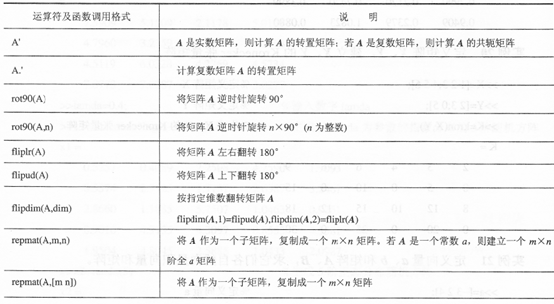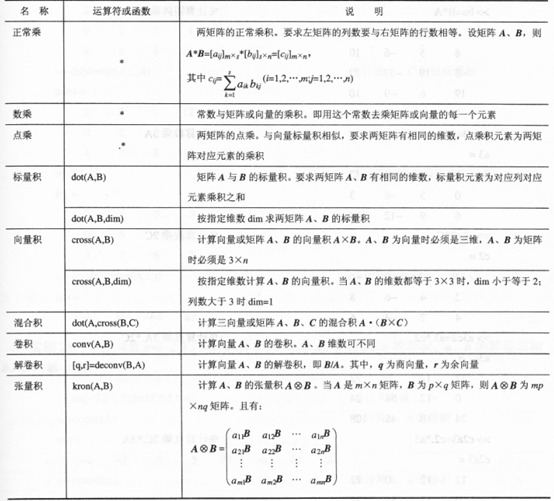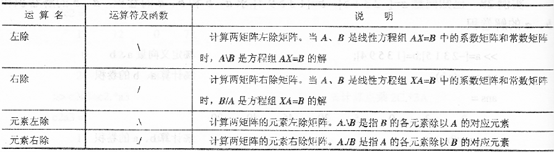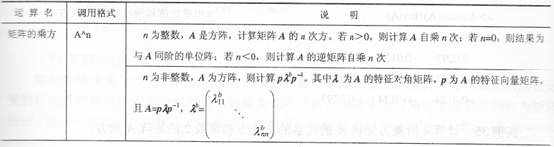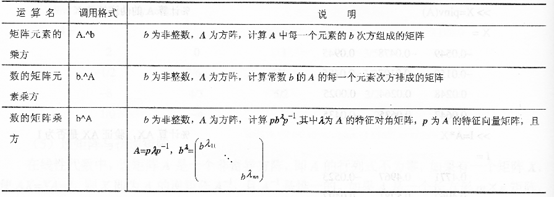计算矩阵的行列式、秩和迹： det(A): 计算方阵A的行列式 rank(A): 计算方阵A的秩 trace(A): 计算方阵A的迹
计算矩阵的特征值与特征向量： eig(A): 计算n阶方阵A的特征值 [U,E]=eig(A): 计算n阶方阵A的特征值和特征向量（特征分解），其中X矩阵的每一列对应一个特征向量。
1.2多项式运算
1.2.1计算多项式的值与根：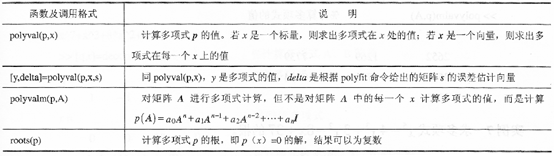1.2.2多项式的运算：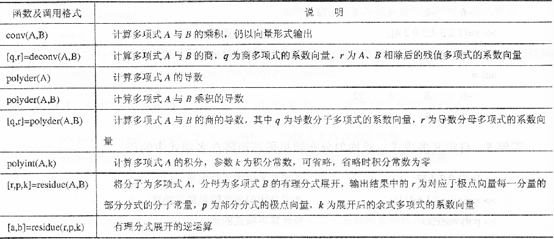1.2.3多项式的拟合与插值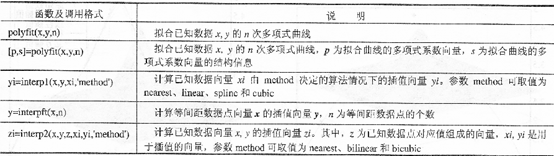1.3数理统计
1.3.1数据的最大、最小值与排序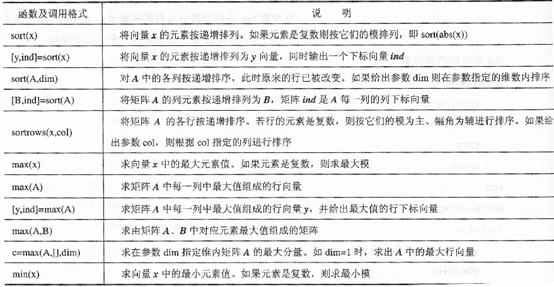1.3.2求和、乘积与差分  sum(x): 求向量x所有元素之和。  sum(A): 求矩阵A的各列元素和。  prod(x): 求向量x中各元素的乘积。  prod(A): 求向量A中各列元素的乘积向量。  diff(x): 求向量x的差分向量，即相邻两元素之差组成的向量。  diff(A): 求矩阵A的列差分矩阵，即求列相邻两元素之差组成的矩阵.
1.3.3平均值、中值与标准差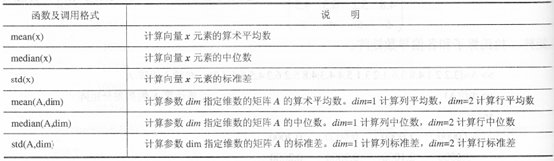1.3.4协方差与相关系数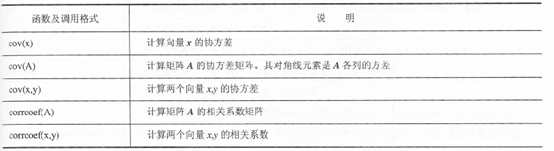1.3.5数据取整 ceil：向上取整 floor：向下取整 fix：向零取整 round：四舍五入 mod、rem：取余数处理 abs(x)：对数据取模 angle(x)：对数据取相位 phase(x)：对数据取相位并解卷绕 real(x)：对数据取实部 imag(x)：对数据取虚部
展开全文线性代数
• 文章目录一.MATLAB基本运算说明二.面向复数的计算特点1.基础知识2.对复数的基本操作3.复数的开方问题二.面向数组1....MATLAB面向复数设计，其所有运算都定义在复数域上，所以对于方根运算运算只...


文章目录
一.MATLAB基本运算说明二.面向复数的计算特点1.基础知识2.对复数的基本操作3.复数的开方问题
二.面向数组1.数组的输入形式2.对矩阵中的元素进行并行操作3.利用数组运算，实现函数可视化4.实现矩阵之间的点乘

一.MATLAB基本运算说明
MATLAB的基本运算符如示：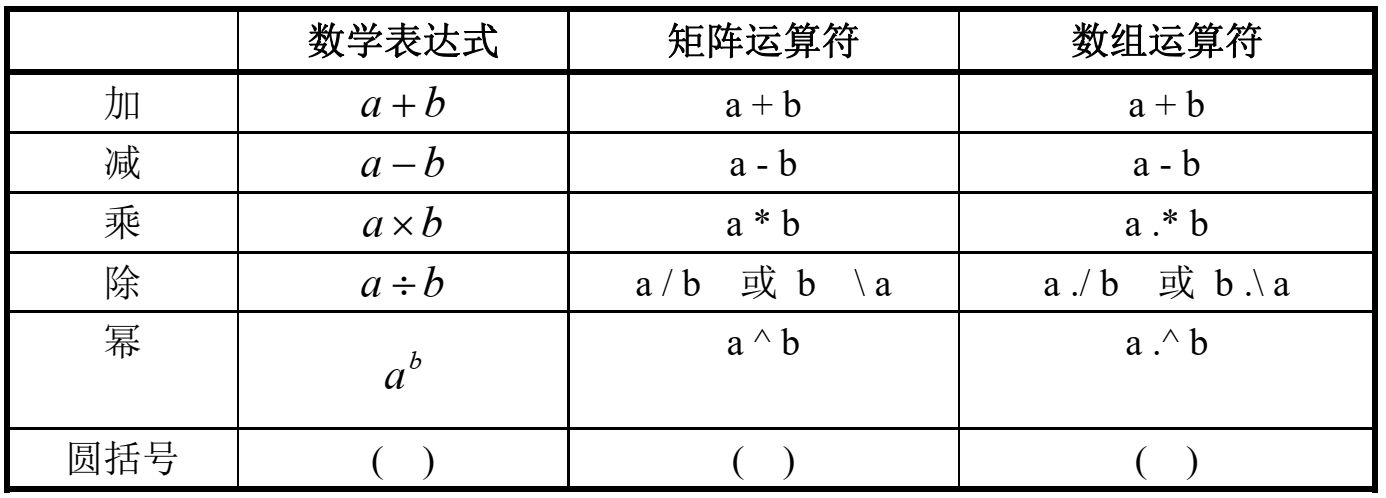求e的x次方：exp(x)

MATLAB面向复数设计，其所有运算都定义在复数域上，所以对于方根运算，运算只返回一个“主解”，所以要得到复数的全部方根，必须编写专门程序。MATLAB面向矩阵/数组设计，所以标量都被看作（1X1）的矩阵/数组。数组运算的“乘、除、幂”规则与相应矩阵运算根本不同。前者的算符比后者多一个“小 黑点”。MATLAB 用左斜杠或右斜杠分别表示“左除”或“右除”运算。对标量而言，“左除” 和“右除”的作用结果相同。但对矩阵来说，“左除”和“右除”将产生不同的结果。
二.面向复数的计算特点
1.基础知识
MATLAB 的所有运算都是定义在复数域上的。这样设计的好处是：在进行运算时，不 必像其他程序语言那样把实部、虚部分开处理。为描述复数，虚数单位用预定义变量 i 或 j 表示。 所以，变量i j都是预定义变量，不可以再被赋值：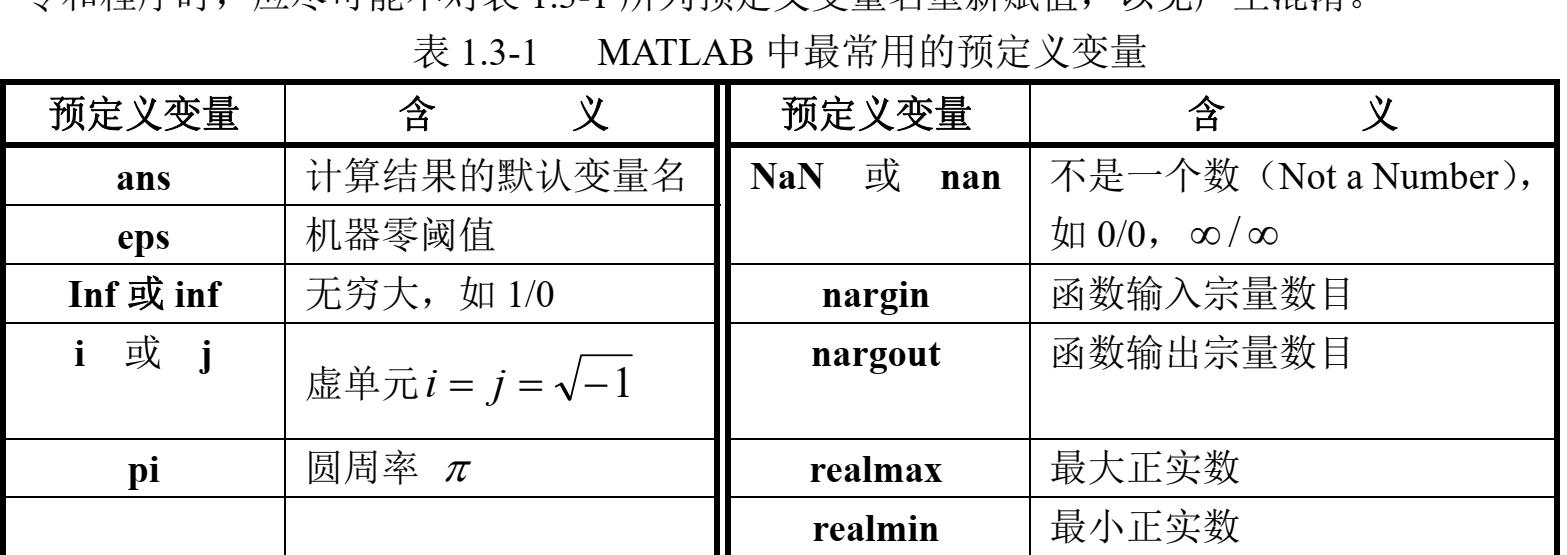2.对复数的基本操作
所以，既然运算都是定义在复数上的，就衍生出了对复数操作的函数：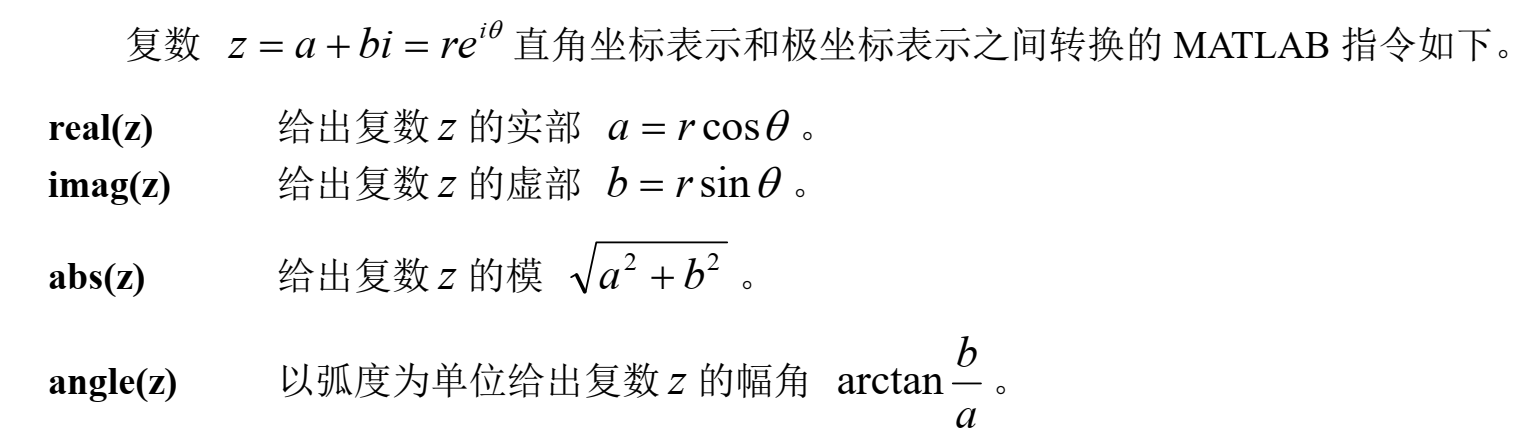将辐角转换为以°为单位：angle(z)*180/pi

3.复数的开方问题分三种方法实现，看看哪种才是准确的

1.先试着直接计算x=(-8)^(1/3) 得出结果如下：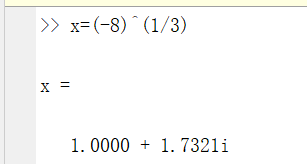显然不是我们预期的结果！！！得到的只是处于第一象限的方根！！！
2.利用解多项式的方法求出 构建多项式x^3-(-8)，然后通过解多项式来得到(-8)^(1/3)的全部解（基于复数域）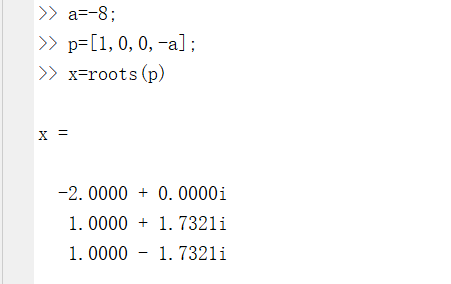可以得出基于复数域的全部解，第一个解-2.0000 + 0.0000i就是实数解-2。 解释一下如何构建多项式以及如何得到多项式的根：

MATLAB表示多项式为包含由下降幂排列的系数的行向量。 例如，方程式: P(x)=4x3-5x2+3x-7 MATLAB中：p=[4,-5,3,-7] 再通过：x=roots( p )就可以得出多项式的根 根会以数组的形式存储在变量x中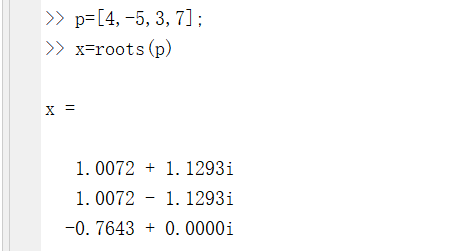3.通过图形表示 基于求解多项式根的前提，将三个解在坐标中表示出来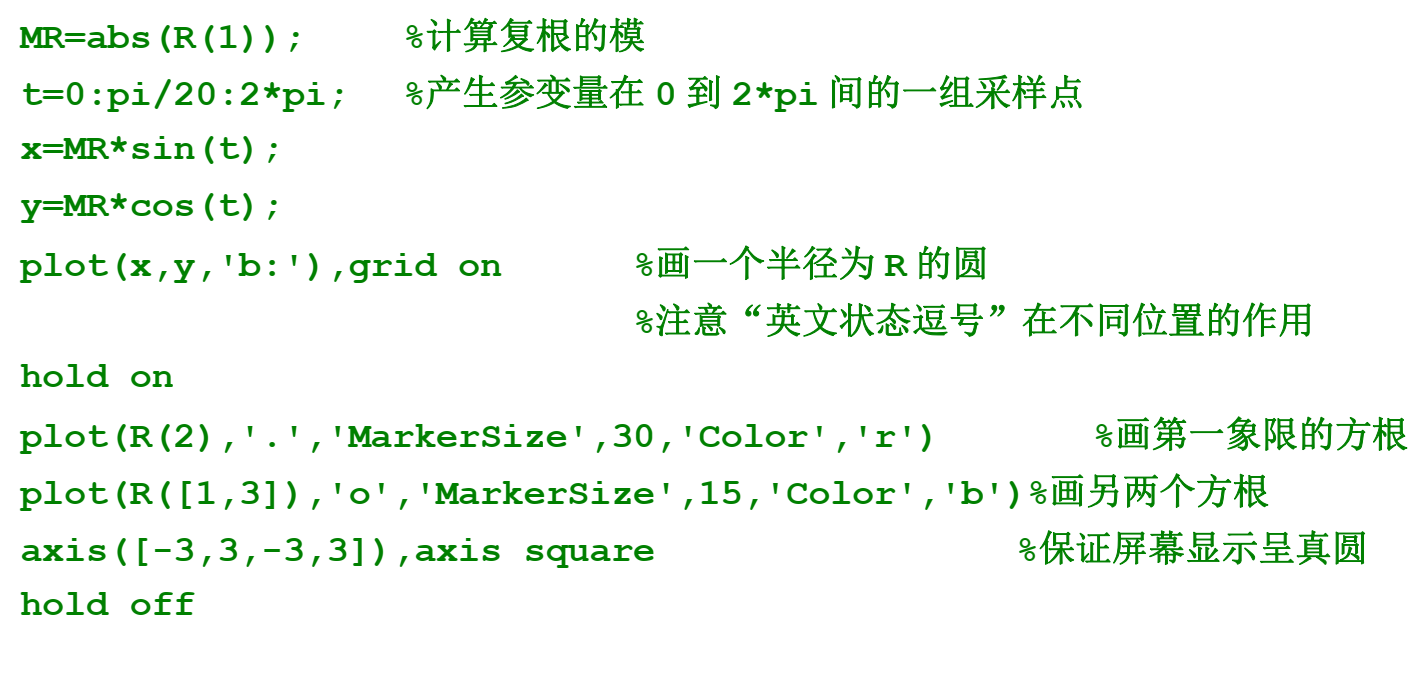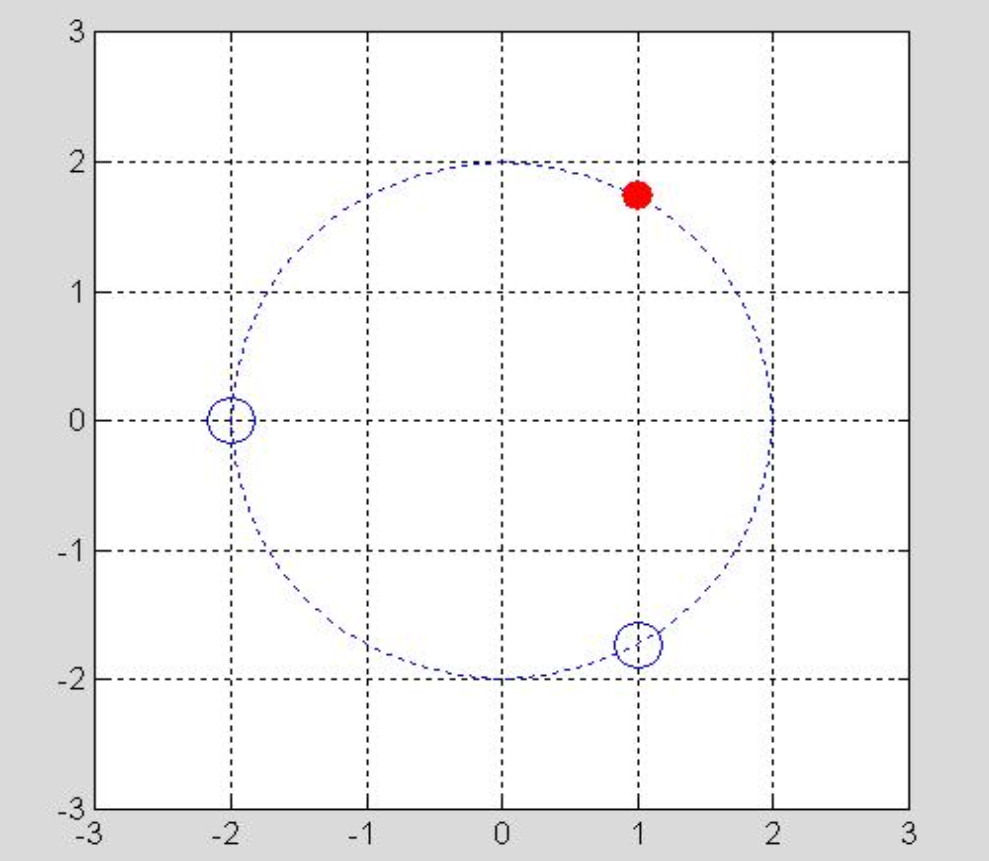二.面向数组
在MATLAB中，标量数据被看作1X1的数组数据，所有的数据都存放在适当大小的数组中。为了加快计算速度，MATLAB对以数组形式存储的数据设计了俩种基本运算：
数组运算矩阵运算
1.数组的输入形式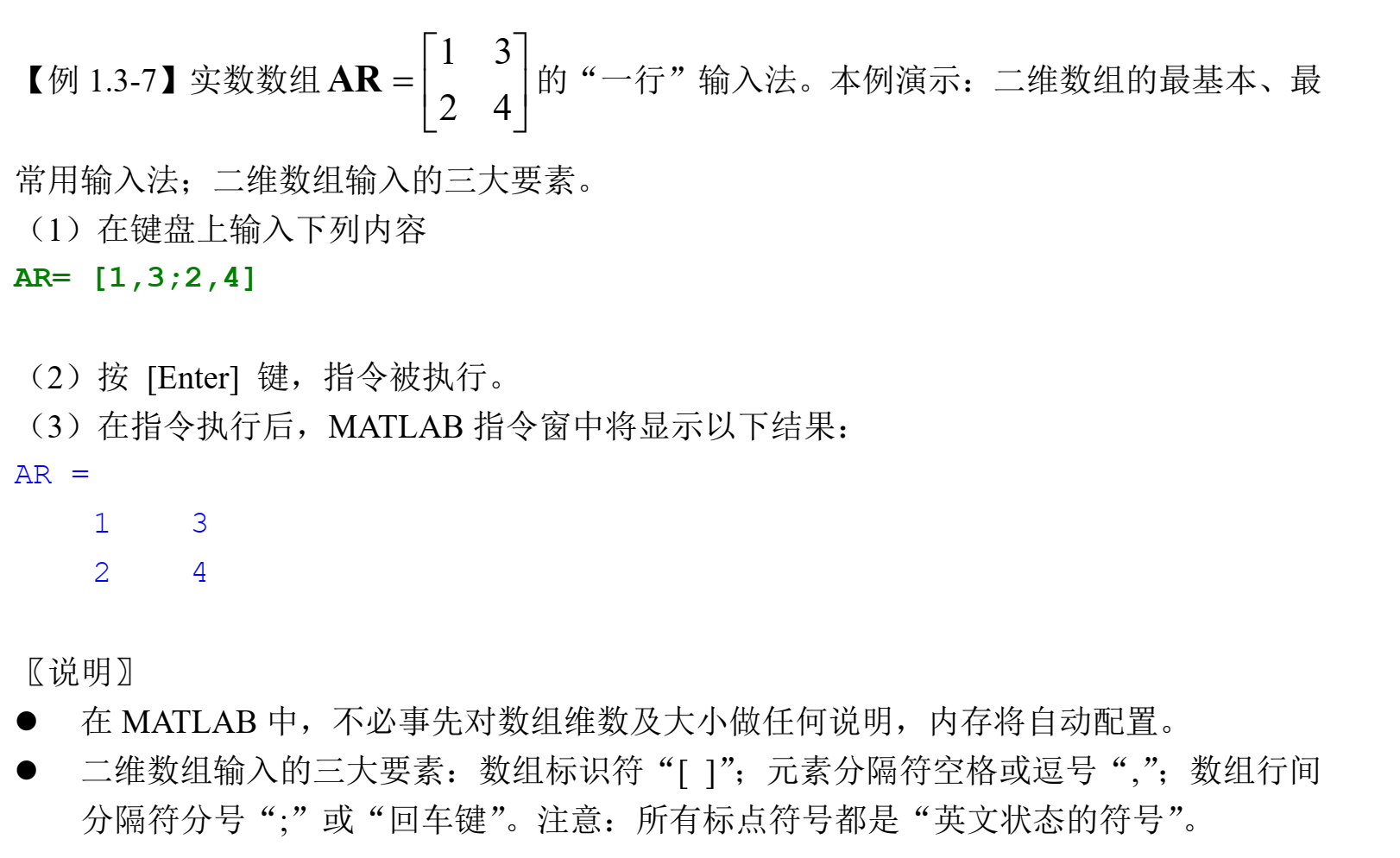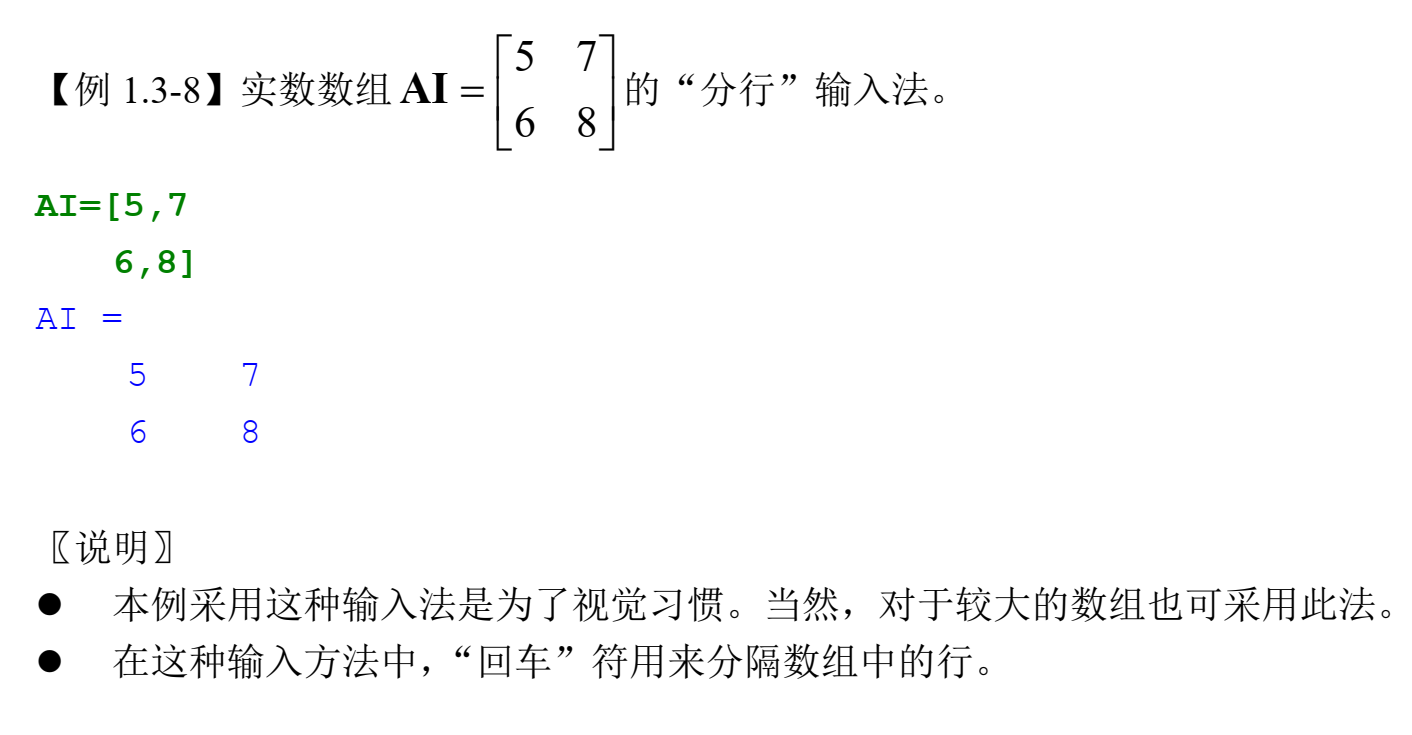2.对矩阵中的元素进行并行操作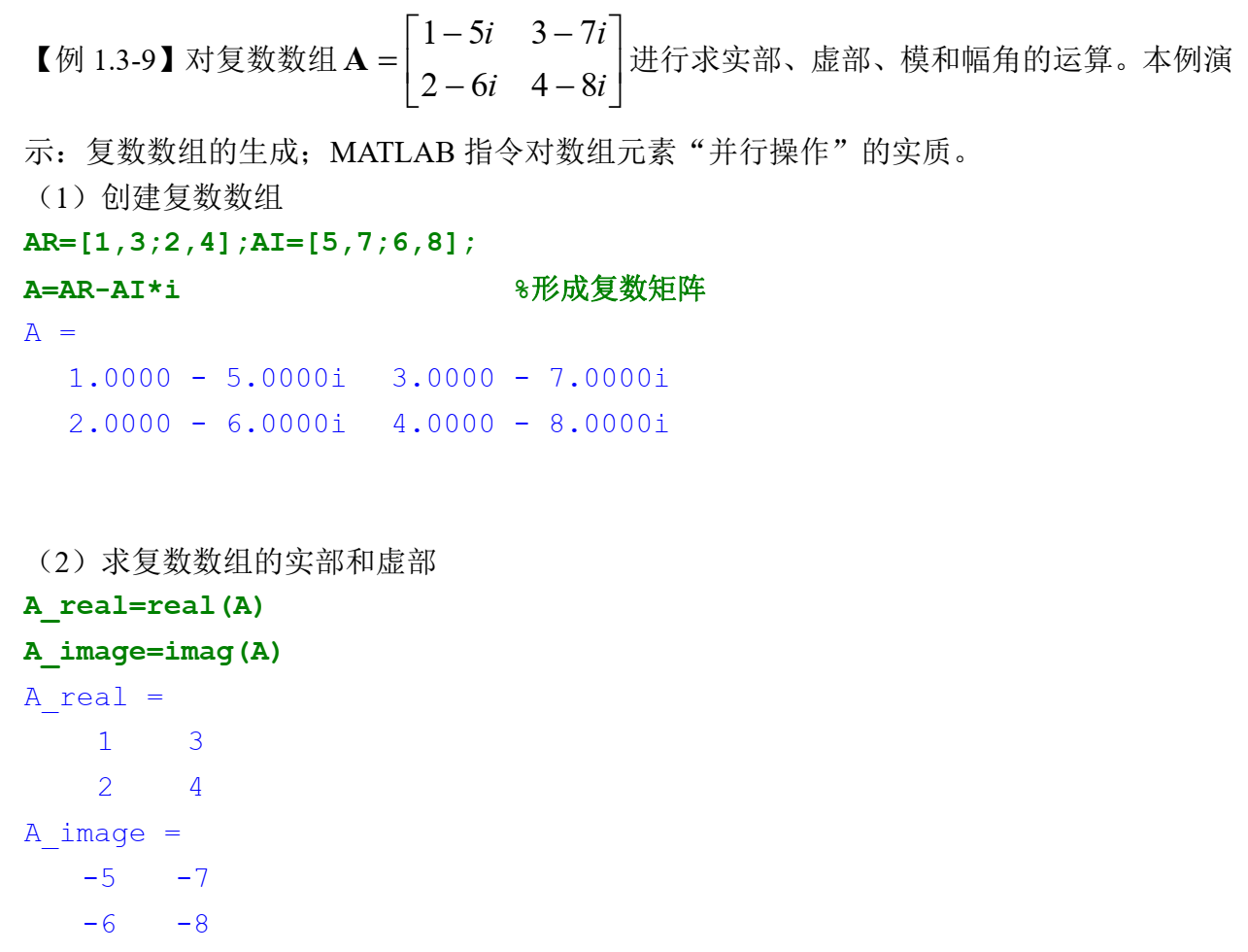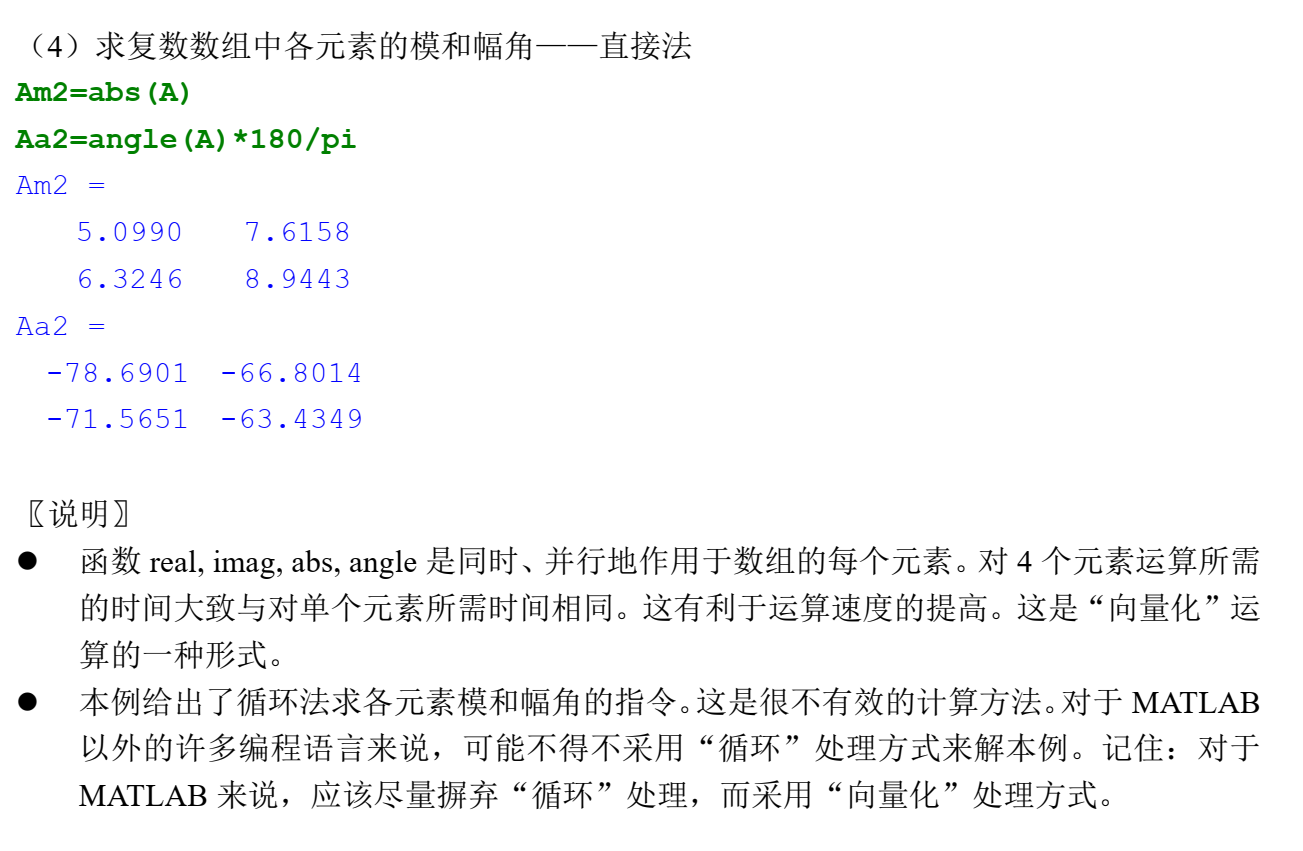3.利用数组运算，实现函数可视化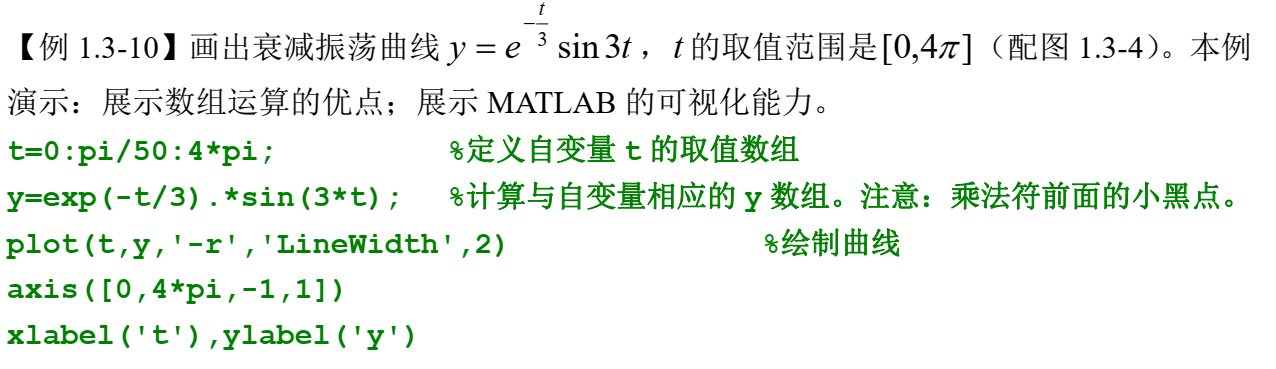最终得出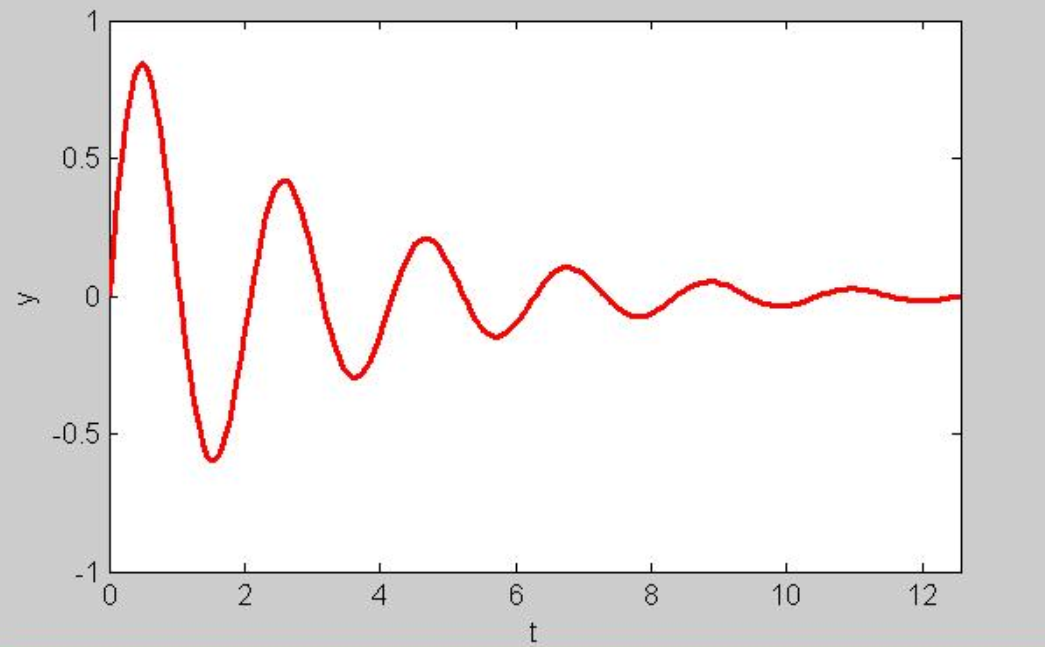需要注意的有：
t=0:pi/50:4*pi相当于创建了一个数组，数组范围为从0到4*pi，元素间距为pi/50。y=exp(-t/3).*sin(3*t)中“.*”符号表示：乘法是在俩个数组相同位置上的元素间进行的，这样才可以做到t于y的一一对应。
4.实现矩阵之间的点乘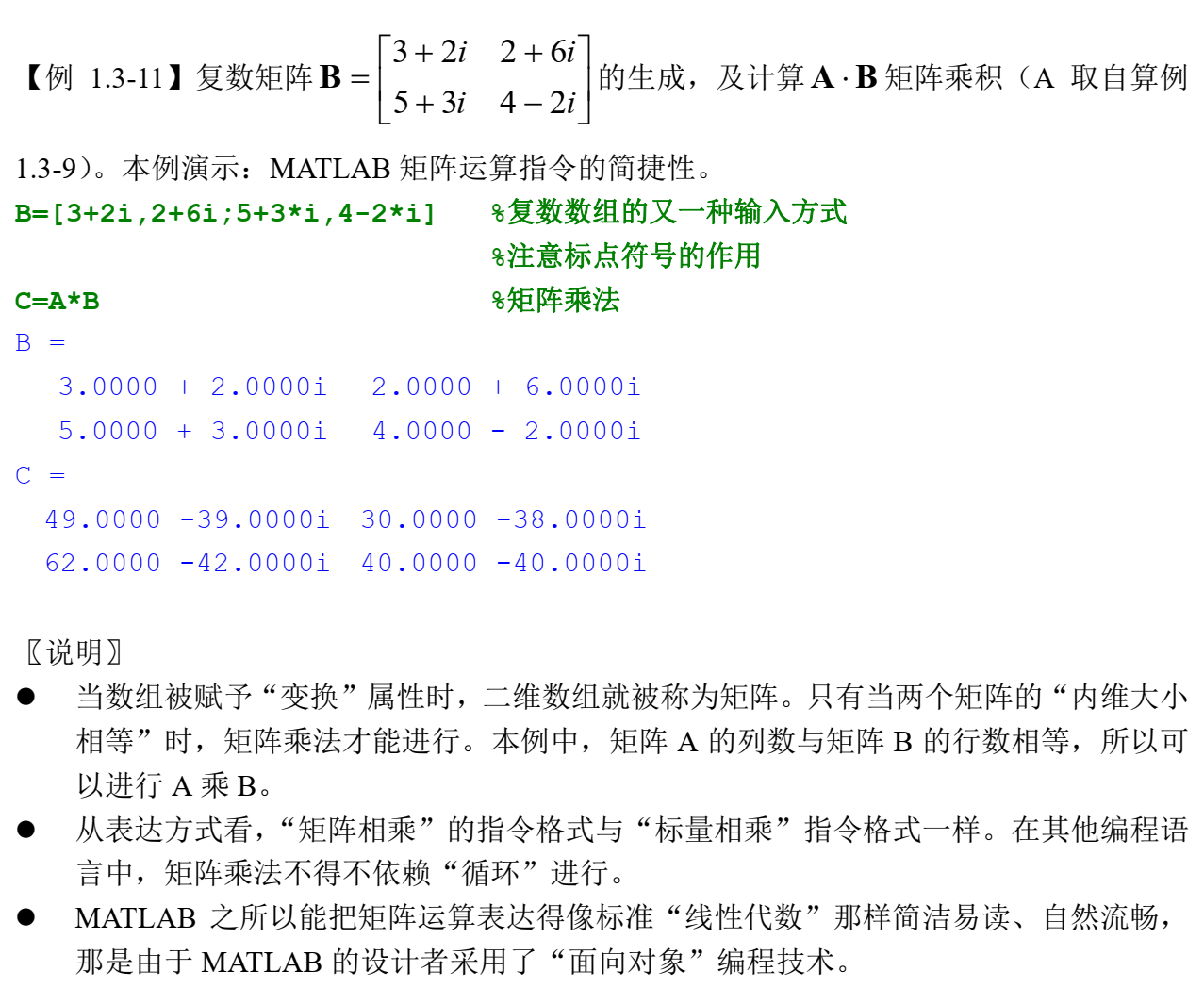展开全文• 今天遇到一个问题，有一个4333*1的**complex double 矩阵**，进行相关运算时候，发现matlab运行出不来结果，于是就开始着手解决这个问题，后来参考很多资料，总结了两种方案，比较得出第二种方案更佳： 首先思路都...complex double
• C++实现复数矩阵求逆 matlab inv一、引言二、原理2.1 实数矩阵求逆2.2 复数矩阵求逆三、代码四、测试 一、引言 之前偶尔一次有用到将matlab转为C++语言的需求，其中matlab有一个inv函数可以非常方便的求矩阵的逆，...c++ 矩阵求逆
• 一、前言 最近在企业实习，花了三个星期的时间肝了一个室内定位算法（雏形，还未优化），实现matlab和C的联写、联调，其难点...谨以此连载文档记录一下算法开发的关键--复数矩阵运算。 网上关于C语言实现矩阵运算的dec语言 指针 算法
• [x x] >> x=[4;5] x = 4 5 >> [x x] ans = 4 4 5 5 >> x=[2,3] x = 2 3 >> [x x] ans = 2 3 2 3 数组、矩阵运算 % 数组运算 % 两个...
• ## MATLAB01:基本的数学运算与矩阵运算

万次阅读 多人点赞 2019-11-12 17:22:52
MATLAB01:基本的数学运算与矩阵运算MATLAB基本语法变量变量名保留变量不适合做变量名变量不应当覆盖内置函数MATLAB的调用优先级变量类型数字型变量的显示格式MATLAB命令行使用MATLAB进行数字运算使用MATLAB计算数学...
• ## Matlab矩阵运算

千次阅读 2014-09-11 10:52:20
Matlab 矩阵运算 说明：这一段时间用Matlab做了LDPC码的性能仿真，过程中涉及了大量的矩阵运算，本文记录了Matlab中矩阵的相关知识，特别的说明了稀疏矩阵和有限域中的矩阵。Matlab的运算是在...
• MATLAB基本语法 变量 1.MATLAB中的变量不需要声明 2.使用 “ = ” 为变量赋值 变量名 1.与大多数编程语言相同，MATLAB中的变量名是大小写敏感的（也就是说大写A与小写a所代表的含义是不一样的） ...复数算子
• MATLAB矩阵合并及相关运算 1、matlab允许向量（和矩阵）合并，且matlab提供了两种合并方式，[a,b]和[a;b]，两者的结果是不一样的。 a=rand(2,3)； b=rand(2,3)； c=[a;b]； d=[a,b]； c的结果是将b整体合并到a 的...
• julia矩阵运算 Julia| 复数 (Julia | Complex Numbers) The syntax to represent the complex number in Julia is: 在Julia中表示复数的语法为： Syntax: 句法： A+Bim Here, A and B are the values, and im...线性代数 cuda xcode mac
• 复数矩阵的加/减/乘运算涉及到其复数元胞（cell）的相加减运算，由于complex.h头文件中只给出了复数乘法运算，故而复数的加减运算函数需要自己定义功能。 二、矩阵加/减法运算复数矩阵复数矩阵之间的加/减法...线性代数 算法 c语言
• 复数复数运算 | matlab可视化 matlab2018a 01 复数 MATLAB中，复数单位通常用 1i来表示，例如z = 1 + 1i。这里需要说明的是，i也可以用来表示复数单位，但是由于i通常用作循环的变量，所以MATLAB建议定义复数时...
• 如图这样的txt文档，我该如何导入到MATLAB的工作区，形成数组，然后对这些数进行运算。 如下是我找到的一段代码 ` clc; clear; fin=fopen('222.txt','r'); result={}; while feof(fin)==0 str=fgetl...开发语言
• 另，这将也是我此连载文章的系列精品之一，矩阵运算的功能越来越复杂，但是笔者全部分模块进行，化繁为简，化整为零，所以需要调用的小函数越来越多，读者遇到不熟悉的函数可以随时Jump到（一）、（二）、（三）、...指针 算法 c语言 线性代数
• 1、行向量的定义 rowvec = [1.2 3 56]; 2、列向量的定义 colvec = [1.2;3;56]; 3、逐个元素的分配矩阵 B(1,1) = 1 ; B(1,2) = 2 ;...注意：矩阵的下标从1开始计数 ...4、矩阵的输入 ...直接输入矩阵时候，...向量 LU分解
• 二、Matlab中最基本的向量与矩阵运算知识 1、对向量的操作 1）向量的基本运算： 定义一个向量 创建一个列向量，元素与元素之间用分号(;)隔开，如 a = [2; 1; 4]; 创建一个行向量，元素与元素之间用空格(space)或者...线性代数
• ## matlab求复数的模

千次阅读 2019-10-15 14:26:29
假设有一个举证 a=[1+2i;2+3i;2+4i;1+4i] a = 1.0000 + 2.0000i 2.0000 + 3.0000i 2.0000 + 4.0000i 1.0000 + 4.0000i 用下列方式求模，结果如下： sqrt(a.^2) ans = 1.0000 + 2.0000i ...1.0000 + 4.000...
• 在这一部分将会介绍基本组成部分，数值，符号，函数，等等 ...是指令输入提示符，是自动生成的，也可以称为运算提示符，表示MATLAB处于准备就绪状态，如在提示符之后输入一条命令或者一段程序之...数据类型
• 1. 矩阵运算和操作函数 cond 矩阵的条件数值 condest 1-范数矩阵条件数值 det 矩阵的行列式值 eig 矩阵的特征值 inv 矩阵的逆 norm 矩阵的范数值 normest 矩阵的2-范数值 rank 矩阵的秩 orth 矩阵的正交...
• y1=a.*b; 这里，假如a与b都是matlab复数数组。 这个点乘就很有迷惑性。 这个点乘只是在数组层面。 而其中的复数乘，还是叉乘。
• 矩阵的分解函数cholCholesky分解cholinc稀疏矩阵的不完全Cholesky分解lu矩阵LU分解luinc稀疏矩阵的不完全LU分解qr正交三角分解svd奇异值分解gsvd一般奇异值分解schur舒尔分解在MATLAB中线性方程组的求解主要基于四种...
• 今天先来谈谈MATLAB中的矩阵运算。 一、矩阵输入 矩阵的输入我也理解的不太透彻，大致形式为a=[1,2,3;4,5,6;7,8,9];其中‘，’表示前后的东西在一行，也可以被空格替换，之所以这么表达，是因为逗号或空格前后也...
• 1、matlab允许向量（和矩阵）合并，且matlab提供了两种合并方式，[a,b]和[a;b]，两者的结果是不一样的。 a=rand(2,3)； b=rand(2,3)； c=[a;b]； d=[a,b]； c的结果是将b整体合并到a 的下边，而d的结果是整体将b合并......

# matlab复数矩阵运算matlab 订阅